Home
Hostname: page-component-55b6f6c457-ln9sz Total loading time: 0.24 Render date: 2021-09-25T10:48:38.824Z Has data issue: true Feature Flags: { "shouldUseShareProductTool": true, "shouldUseHypothesis": true, "isUnsiloEnabled": true, "metricsAbstractViews": false, "figures": true, "newCiteModal": false, "newCitedByModal": true, "newEcommerce": true, "newUsageEvents": true }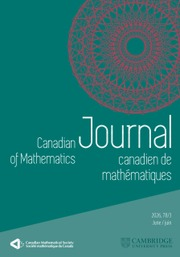Canadian Journal of Mathematics

# Hankel Operators on Pseudoconvex Domains of Finite Type in ℂ2

Published online by Cambridge University Press:  20 November 2018

## Abstract

HTML view is not available for this content. However, as you have access to this content, a full PDF is available via the ‘Save PDF’ action button.

The aim of this paper is to study small Hankel operators$h$ on the Hardy space or on weighted Bergman spaces,where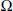$\Omega$ is a finite type domain in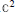${{\mathbb{C}}^{2}}$ or a strictly pseudoconvex domain in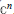${{\mathbb{C}}^{n}}$ . We give a sufficient condition on the symbol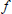$f$ so that$h$ belongs to the Schatten class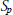${{S}_{p}}$ ,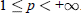$1\,\le \,p\,<\,+\infty$ .

## Keywords

Type
Research Article
Information
Canadian Journal of Mathematics , 01 June 1998 , pp. 658 - 672

## References

[AFP] Arazy, A., Fisher, S. and Peetre, J., Hankel operators on weighted Bergman spaces. Amer. J. Math. 111(1988), 9891054.Google Scholar
[BL1] Beatrous, F. and Li, S-Y., On the boundedness and compactness of operators of Hankel type. J. Funct. Anal. 111(1993), 350379.Google Scholar
[BL2] Beatrous, F., Trace Ideals Criteria for Operators of Hankel type. Illinois J. Math. 39(1995), 723754.Google Scholar
[B] Boas, H., The Szegö projection: Sobolev estimates in regular domains. Trans. Amer. Math. Soc. 300(1987), 109132.Google Scholar
[B1] Bell, S., A duality theorem for harmonic functions. Michigan Math. J. 29(1982), 123128.Google Scholar
[B2] Bell, S., Biholomorphic mappings and the δ -problem. Ann. of Math. 114(1981), 103114.Google Scholar
[BCG] Bonami, A., Der-Chen Chang and Grellier, S., Commutation properties and Lipschitz estimates for the Bergman and Szegö projection. Math Z. 223(1996), 275302.Google Scholar
[BPS1] Bonami, A., Peloso, M. and Symesak, F., Powers of the Szegö kernel and Hankel operators on Hardy spaces. Preprint.Google Scholar
[BPS2] Bonami, A., Factorization theorems for Hardy spaces and Hankel operators. In preparation.Google Scholar
[Ca] Catlin, D., Estimates of invariant metrics on pseudoconvex domains of dimension two. Math.Z. 200(1989), 429466.Google Scholar
[CR] Coifman, R. and Rochberg, R., Representation theorem for holomorphic and harmonic functions in Lp. Astérisque 77(1980), 165.Google Scholar
[CRW] Coifman, R., Rochberg, R. and G.Weiss, Factorization theorems for Hardy spaces in several variables. Ann. of Math. 103(1976), 611635.Google Scholar
[CW] Coifman, R. et Weiss, G., Analyse harmonique non commutative sur certains espaces homog`enes. Lecture Notes in Math. 242, Springer-Verlag, 1971.CrossRefGoogle Scholar
[Co] Coupet, B., D´ecomposition atomique des espaces de Bergman. Indiana Math. J. 38(1989), 917941.Google Scholar
[FR] Feldman, M. and Rochberg, R., Singular value estimates for commutators and Hankel operators on the unit ball and the Heisenberg group. Lecture Notes in Pure and Appl. Math. 122 (Analysis and partial differential equations), Marcel Dekker, New York, 1990.Google Scholar
[GK] Gohberg, I. and Krein, M.G., Introduction to the theory of non-self adjoint operators. Trans. Math. Monograph. 18, Amer. Math. Soc., Providence, RI, 1969.Google Scholar
[J] Janson, S., On functions with conditions on the mean oscillation. ArkMat. 14(1976), 189190.Google Scholar
[KL1] Krantz, S. and Li, S-Y., On decomposition theorems for Hardy spaces on domains in Cn and applications. J. Fourier Anal. App. 2(1995), 65107.Google Scholar
[KL2] Krantz, S., Hardy classes, integral operators, and duality on spaces of homogeneous type. Preprint.Google Scholar
[KLR] Krantz, S., Li, S-Y. and Rochberg, R., The effect of boundary geometry on Hankel operators belonging to the trace ideals of Bergman spaces. Preprint.Google Scholar
[KLLR] Krantz, S., Li, S-Y., Lin, P. and Rochberg, R., The effect of regularity on the singular number of Friedrichs operators on Bergman spaces. Michigan Math. J. 43(1996), 337–348.Google Scholar
[L] Li, H., Schatten class Hankel operators on Bergman space of strongly pseudoconvex domain. Integral Equations Operator Theory 19(1994), 458–476.CrossRefGoogle Scholar
[NSW] Nagel, A., Stein, E. and Wainger, S., Balls and metrics defined by vector fields I: basic properties. Acta Math. 155(1985), 103–147.CrossRefGoogle Scholar
[NRSW] Nagel, A., Rosay, J-P., Stein, E. and Wainger, S., Estimates for the Bergman and the Szegö kernel in C2 . Ann. of Math. 129(1989), 113–149.CrossRefGoogle Scholar
[Pell] Peller, V. V., Hankel operators of class Cp and their application (rational approximation, Gaussian processes, the problem of majorizing operators). Math. of the USSR-Sbornik 41(1982), 443–479.CrossRefGoogle Scholar
[Pelo] Peloso, M., Hankel operators on weighted Bergman spaces on strictly pseudoconvex domains. Illinois J. Math. 38(1995), 223–249.Google Scholar
[RS1] Rochberg, R. and Semmes, S., A decomposition theorem for BMO and application. J. Funct. Anal. 67(1986), 228–263.CrossRefGoogle Scholar
[RS2] Rochberg, R. and Semmes, S., Nearly weakly orthonormal sequences, singular value estimates and Calderon-Zygmund operators. J. Funct. Anal. 86(1989), 237–306.CrossRefGoogle Scholar
[S1] Symesak, F., Décomposition atomique des espaces de Bergman. Publ. Mat. 39(1995), 285–299.CrossRefGoogle Scholar
[S2] Symesak, F., Hankel operators on complex ellipsoids. Illinois. J. Math. 40(1996), 632–647.Google Scholar
[Zha] Zhang, G., Hankel operators on Hardy spaces and Shatten classes. Chinese Ann. Math. Ser. B 12(1991), 282–294.Google Scholar
[Zhe] Zheng, D., Shatten class Hankel operators on Bergman spaces. Integral Equations Operator Theory 13(1990), 442–459.CrossRefGoogle Scholar
[Zhu] Zhu, K., Operator Theory in function spaces. Decker, New York, 1990.Google ScholarYou have Access
2
Cited by

# Send article to Kindle

Note you can select to send to either the @free.kindle.com or @kindle.com variations. ‘@free.kindle.com’ emails are free but can only be sent to your device when it is connected to wi-fi. ‘@kindle.com’ emails can be delivered even when you are not connected to wi-fi, but note that service fees apply.

Find out more about the Kindle Personal Document Service.

Hankel Operators on Pseudoconvex Domains of Finite Type in ℂ2
Available formats
×

# Send article to Dropbox

To send this article to your Dropbox account, please select one or more formats and confirm that you agree to abide by our usage policies. If this is the first time you use this feature, you will be asked to authorise Cambridge Core to connect with your <service> account. Find out more about sending content to Dropbox.

Hankel Operators on Pseudoconvex Domains of Finite Type in ℂ2
Available formats
×

# Send article to Google Drive

To send this article to your Google Drive account, please select one or more formats and confirm that you agree to abide by our usage policies. If this is the first time you use this feature, you will be asked to authorise Cambridge Core to connect with your <service> account. Find out more about sending content to Google Drive.

Hankel Operators on Pseudoconvex Domains of Finite Type in ℂ2
Available formats
×
×